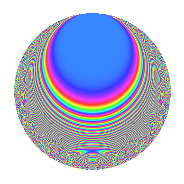# Properties

 Label 473.2.aLevel 473 Weight 2 Character orbit a Rep. character $$\chi_{473}(1,\cdot)$$ Character field $$\Q$$ Dimension 35 Newforms 7 Sturm bound 88 Trace bound 3

# Related objects

## Defining parameters

 Level: $$N$$ = $$473 = 11 \cdot 43$$ Weight: $$k$$ = $$2$$ Character orbit: $$[\chi]$$ = 473.a (trivial) Character field: $$\Q$$ Newforms: $$7$$ Sturm bound: $$88$$ Trace bound: $$3$$ Distinguishing $$T_p$$: $$2$$, $$3$$

## Dimensions

The following table gives the dimensions of various subspaces of $$M_{2}(\Gamma_0(473))$$.

Total New Old
Modular forms 46 35 11
Cusp forms 43 35 8
Eisenstein series 3 0 3

The following table gives the dimensions of the cuspidal new subspaces with specified eigenvalues for the Atkin-Lehner operators and the Fricke involution.

$$11$$$$43$$FrickeDim.
$$+$$$$+$$$$+$$$$8$$
$$+$$$$-$$$$-$$$$11$$
$$-$$$$+$$$$-$$$$9$$
$$-$$$$-$$$$+$$$$7$$
Plus space$$+$$$$15$$
Minus space$$-$$$$20$$

## Trace form

 $$35q + q^{2} + 2q^{3} + 31q^{4} - 4q^{5} - 8q^{6} + 8q^{7} - 15q^{8} + 41q^{9} + O(q^{10})$$ $$35q + q^{2} + 2q^{3} + 31q^{4} - 4q^{5} - 8q^{6} + 8q^{7} - 15q^{8} + 41q^{9} - 14q^{10} - 3q^{11} - 12q^{12} - 2q^{13} - 16q^{14} - 22q^{15} + 35q^{16} - 14q^{17} - 19q^{18} + 2q^{20} + 20q^{21} + 3q^{22} - 10q^{23} - 8q^{24} + 31q^{25} - 2q^{26} - 10q^{27} - 8q^{28} + 10q^{29} + 24q^{30} - 2q^{31} - 39q^{32} + 2q^{33} - 38q^{34} + 12q^{35} + 39q^{36} - 4q^{37} - 4q^{38} - 8q^{39} - 50q^{40} + 18q^{41} + 32q^{42} + q^{43} - 3q^{44} - 2q^{45} + 12q^{46} - 36q^{47} - 24q^{48} + 79q^{49} + 35q^{50} + 18q^{52} - 22q^{53} - 16q^{54} - 8q^{56} - 20q^{57} - 34q^{58} - 6q^{59} - 4q^{60} - 10q^{61} + 52q^{62} - 68q^{63} - 5q^{64} - 56q^{65} - 16q^{66} - 6q^{67} - 14q^{68} - 26q^{69} + 12q^{70} + 18q^{71} - 43q^{72} + 22q^{73} + 54q^{74} + 12q^{75} - 8q^{76} + 4q^{77} - 12q^{78} + 40q^{79} + 10q^{80} + 59q^{81} - 58q^{82} - 8q^{83} + 48q^{84} - 36q^{85} - 7q^{86} - 8q^{87} - 9q^{88} - 8q^{89} + 2q^{90} + 56q^{91} + 16q^{92} + 14q^{93} - 8q^{94} - 16q^{95} + 56q^{96} - 8q^{97} - 7q^{98} - 9q^{99} + O(q^{100})$$

## Decomposition of $$S_{2}^{\mathrm{new}}(\Gamma_0(473))$$ into irreducible Hecke orbits

Label Dim. $$A$$ Field CM Traces A-L signs $q$-expansion
$$a_2$$ $$a_3$$ $$a_5$$ $$a_7$$ 11 43
473.2.a.a $$1$$ $$3.777$$ $$\Q$$ None $$-2$$ $$1$$ $$-1$$ $$0$$ $$+$$ $$+$$ $$q-2q^{2}+q^{3}+2q^{4}-q^{5}-2q^{6}-2q^{9}+\cdots$$
473.2.a.b $$2$$ $$3.777$$ $$\Q(\sqrt{5})$$ None $$1$$ $$-4$$ $$2$$ $$0$$ $$+$$ $$+$$ $$q+\beta q^{2}-2q^{3}+(-1+\beta )q^{4}+(2-2\beta )q^{5}+\cdots$$
473.2.a.c $$2$$ $$3.777$$ $$\Q(\sqrt{5})$$ None $$1$$ $$-2$$ $$2$$ $$-4$$ $$-$$ $$-$$ $$q+\beta q^{2}-2\beta q^{3}+(-1+\beta )q^{4}+2\beta q^{5}+\cdots$$
473.2.a.d $$5$$ $$3.777$$ 5.5.173513.1 None $$-3$$ $$-1$$ $$-4$$ $$-9$$ $$-$$ $$-$$ $$q+(-1-\beta _{3})q^{2}+(-1+\beta _{1}-\beta _{4})q^{3}+\cdots$$
473.2.a.e $$5$$ $$3.777$$ 5.5.38569.1 None $$1$$ $$-3$$ $$-6$$ $$-15$$ $$+$$ $$+$$ $$q+(\beta _{1}+\beta _{3})q^{2}+(-1-\beta _{2}-\beta _{4})q^{3}+\cdots$$
473.2.a.f $$9$$ $$3.777$$ $$\mathbb{Q}[x]/(x^{9} - \cdots)$$ None $$4$$ $$5$$ $$0$$ $$19$$ $$-$$ $$+$$ $$q+\beta _{1}q^{2}+(1+\beta _{4})q^{3}+(1+\beta _{2}-\beta _{3}+\cdots)q^{4}+\cdots$$
473.2.a.g $$11$$ $$3.777$$ $$\mathbb{Q}[x]/(x^{11} - \cdots)$$ None $$-1$$ $$6$$ $$3$$ $$17$$ $$+$$ $$-$$ $$q-\beta _{1}q^{2}+(1-\beta _{8})q^{3}+(1+\beta _{2})q^{4}+\cdots$$

## Decomposition of $$S_{2}^{\mathrm{old}}(\Gamma_0(473))$$ into lower level spaces

$$S_{2}^{\mathrm{old}}(\Gamma_0(473)) \cong$$ $$S_{2}^{\mathrm{new}}(\Gamma_0(11))$$$$^{\oplus 2}$$$$\oplus$$$$S_{2}^{\mathrm{new}}(\Gamma_0(43))$$$$^{\oplus 2}$$